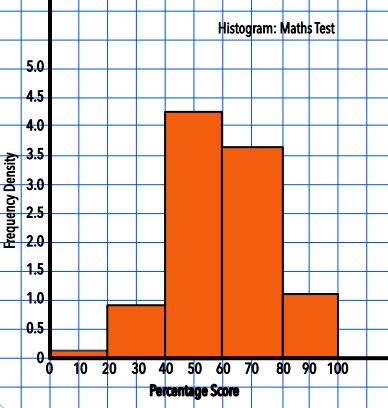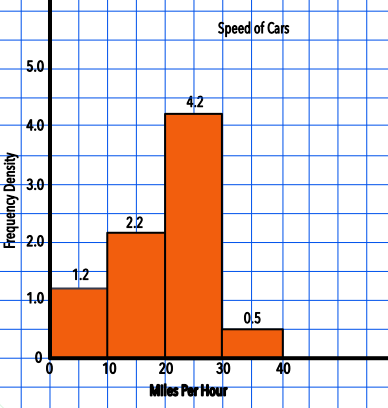Histograms with Equal Intervals

# Histograms with Equal Intervals

GCSE(H),

A Histogram shows the frequency of Grouped Data in Class Intervals.

On a bar chart, the height of the bar gives the frequency. On a histogram, the area of the bar represents the frequency, rather than the height.

The height of each histogram bar is calculated by dividing the frequency by the Class Width. This height is called the Frequency Density. For a histogram with equal intervals, the class width is constant.

The table below shows percentage scores for a maths test. The table shows the Frequency Density and the resultant histogram.

HourFrequencyClass widthFrequency Density
0 < h ≤ 20320 0.15
20 < h ≤ 4018200.90
40 < h ≤ 6085204.25
60 < h ≤ 8073203.65
80 < h ≤ 10023201.15## Examples

1. The speed of cars passing a point on the road was recorded over a period of one hour. The data was plotted on a histogram. From the histogram, below, determine the number of cars that passed the point at more than 30mph.The width of the class interval is 10 (10mph).

The frequency density for 30 < s ≤ 40 is 0.5

0.5 x 10 = 5 cars

2. The table below shows the amount that customers spent at a local shop. A histogram is to be plotted for this data. What is the value of frequency density for the class interval £0 < S ≤ £5?

Amount (P)Frequency
0 < h ≤ 548
5 < h ≤ 1032
10 < h ≤ 1515
15 < h ≤ 2017
20 < h ≤ 258
25 < h ≤ 304

Frequency density = frac(text(frequency))(text(class interval)) = 48 ÷ 5 = 9.6